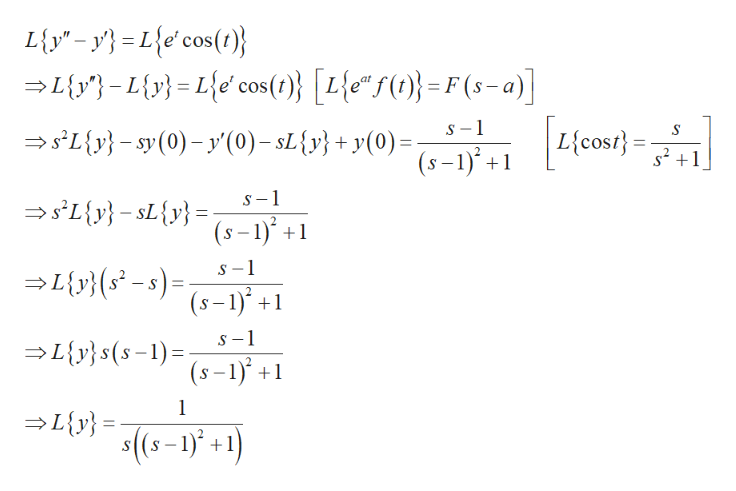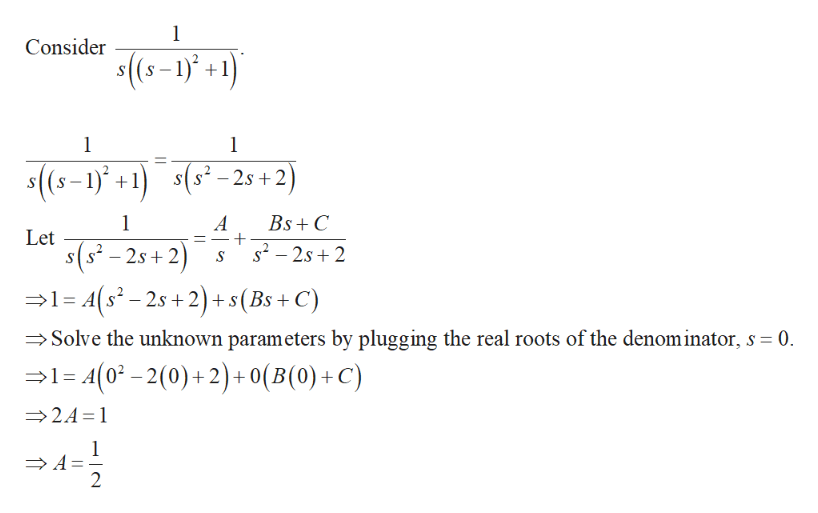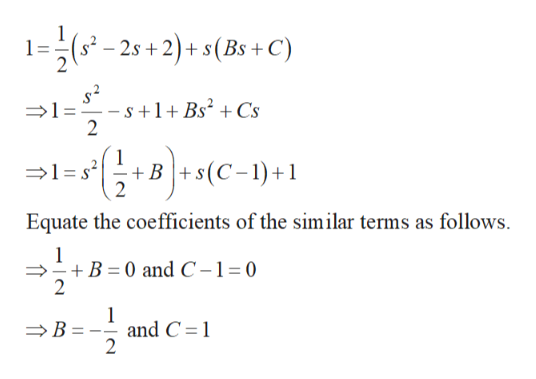# Use the Laplace transform to solve the given initial-value problem.y''-y'=et cos(t),    y(0)=0,    y'(0)=0

Question
48 views

Use the Laplace transform to solve the given initial-value problem.

y''-y'=et cos(t),    y(0)=0,    y'(0)=0

check_circle

Step 1

Take Laplace transform on both the sides as follows.help_outlineImage TranscriptioncloseLiy=y}=L{e'cos(t)} Ly-L Le cos)} [L{&°5(1}= F (5-a)] s1 sLysy (0)'(0)-sL{y}+ y(0)=- (s-1)+1 L{cost) S-1 s'L{y}-sL{v} = (s-1)+1 s-1 _ (s-1)+ s-1 Ly}s(s-1) (s-1 1 L{y} (s-+) fullscreen
Step 2

Take partial fraction on the RHS of the obtained equation.help_outlineImage TranscriptioncloseConsider (s-1)+1) 1 \$(s-1)+1(5-2s+2) 1 A Bs+C Let s(s-2s 2 s2-2s2 1-A(s-2s+2)+ s(Bs + C Solve the unknown parameters by plugging the real roots of the denom inator, s 0 1= 4(0-2(0)+ 2) + 0(B(0) + C) A 24 1 A 2 fullscreen
Step 3

Plug in the solutions to the kno...help_outlineImage Transcriptionclose1=-25+2)+ s(Bs + C) -s +1+ Bs2 +Cs 2 B(C-1)1 Equate the coefficients of the similar terms as follows -B 0 and C-1=0 2 and C 1 2 B fullscreen

### Want to see the full answer?

See Solution

#### Want to see this answer and more?

Solutions are written by subject experts who are available 24/7. Questions are typically answered within 1 hour.*

See Solution
*Response times may vary by subject and question.
Tagged in

### Math